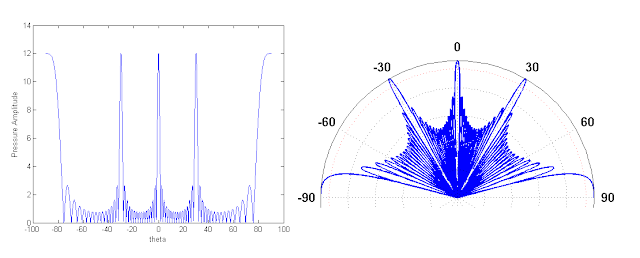## Wednesday, April 8, 2009

### Modeling Grating Lobes in Matlab

For a project for my underwater acoustics class, I am modeling acoustic pressure amplitudes and beam patterns in Matlab. The project involves a continuous line array that we are discretizing in order to calculate the contributing pressure of each point on the array to some arbitrary field point at range r. Basically we are breaking up the line source into n number of elements. When you do this, the spacing of your elements becomes an important factor. If your spacing is equal to, or greater than, one wavelength, you end up with grating lobes. Grating lobes have the same pressure amplitude as your main lobe, but they occur off-nadir and get progressively wider. Each grating lobe has its own associated side lobes.

Below are two examples of what I am talking about. In the first figure, I spaced my elements at a distance equal to 1 wavelength of the transducer. In the second figure, the spacing is 2 wavelengths. In both figures, the main lobe is centered on zero degrees, and you can see that the first side lobe occurs at -13.3 dB. In the first figure the grating lobes start at 90 degrees, and in the second they start at 30. In both cases, the side lobes of the grating lobes also start at -13.3 dB.